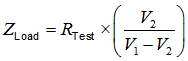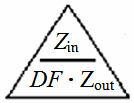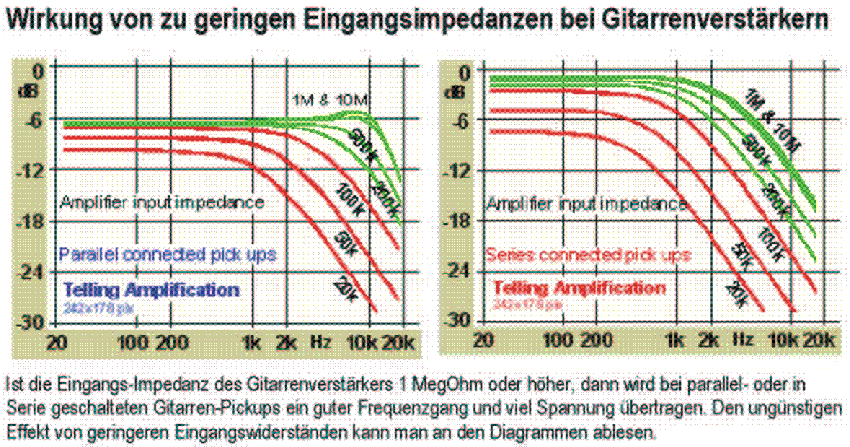Deutsche VersionFinding of input impedance and output impedance
Impedance calculated for audio amplifier, loudspeaker, or microphone

The usual common question is: How to measure impedance?

The question of the input impedance of a microphone or the output impedance of a loudspeaker is nonsense.
There is only the output impedance of a microphone and the input impedance of a loudspeaker.
What is the load resistance of an audio amplifier? That is the loudspeaker.

 In sound engineering there is no Impedance matching or Power matching. In audio we use only high Impedance bridging or Voltage bridging.

'8 Ohm Output' and '150 Ohm Input' - What is that?

Amplifier, Loudspeaker, and Ohms - How do they work together?

 A resistance is a DC resistance, which can be measured with an ohmmeter. If there is a capacitor in the signal path we measure nothing. With a voice coil of a speaker we can measure a DC resistance. With a digital multimeter DMM resistances can be measured easily, but we cannot measure input impedances and output impedances. The capacitance and the resistance build as frequency-dependent form a complex resistance, the so-called impedance Z. The nominal impedance is in electrical engineering and electro-acoustic (audio), the frequency-dependent impedance at the input and / or at the output of an electrical device, which is specified in the middle frequency range at 1 kHz of a technical data sheet. In electrical engineering and acoustics alternating quantities are always described with its effective value (RMS).

Output Impedance Measurement and CalculatorOpen-circuit voltage V1 volts Load resistance Rload ohms Loaded circuit voltage V2 volts ↓ Output Impedance Zsource ohms
 Output impedanceVoltage measurement at the points at OUT:   V1 = Open-circuit voltage (Rload = ∞ Ω, that is without Rload, switch S is open) Rload = Load resistance (Rtest is resistor to measure Ω value) V2 = Loaded circuit voltage with resistor Rload = resistance Rtest Zsource = The output impedance can be calculated

 When the voltage V2 is equal to half of V1, then the measured resistance value Rload (that is Rtest) is equal to the output impedance Zsource.

Zsource = output impedance = source impedance = internal impedance.

Internal resistance of a power amplifier

 "Measuring the output impedance by means of a burden": Suppose there is a 100 watt amplifier. Then the output voltage at half power is P = 50 W = V2 / R.  Loudspeaker impedance = 8 ohms. V = √(P × R) = √ (50 × 8) = 20 volts. (You can also use 10 V.) Give a sine voltage of 1 kHz to the amplifier input, until we get 20 volts at the output. Now we apply the "90% method", that is when we put an output resistance R, until there appear 90% of the open circuit voltage, in this case 18 volts. The internal resistance is then calculated with the 90% method:

 The 90% method Rinternal = R / 9

 At the output fix an oscilloscope, because the wave form should not show any distortion. For example, if R is measured 1 Ohm, then Rinternal = 0.11 Ohm.

Input Impedance Measurement and CalculatorGenerator signal V1 volts Series resistance Rtest ohms Signal after resistor V2 volts ↓ Input impedance Zload ohms
 Input impedanceVoltage measurement at the points IN or at OUT:   V1 = Generator signal voltage (at Rs = 0 Ω, that is without series resistor Rs) Rs = Series resistance (Rtest is resistor to measure Ω value) V2 = Voltage with series resistor Rs = resistance Rtest Zload = The input impedance can be calculated

 When the voltage V2 is equal to half of V1, then the measured resistance value Rs (Rtest) is equal to the input impedance Zload.

Zload = input impedance = load impedance = external impedance = terminator

 The input and output impedance of a four-terminal network can be determined by measuring the alternating current strength in amperes and the AC voltage in volts. The measurement of input impedance typically occurs as follows: The voltage is measured across the input terminals IN. Then, the current in the circuit is done by the device in series with the signal generator. For circuits with high input impedance the current is very small and difficult to measure. R = U / I. Therefore, we choose for the measurement of high-impedance circuits, a better method. It puts a series resistor Rs in the input circuit. First, we measure the input of the device at point IN with V1, the AC voltage, if the resistor Rs = 0 Ohm. Then we measure the RS series resistor, the voltage V2. Then these found valuesV1, Rs and V2 is entered in the above calculator to find the input impedance to be calculated. Search for a suitable measuring resistance value Rs. For typical audio equipment that will be about 10 to 100 kilo-ohms.   You can use the digital voltmeter instead at the measuring point IN and at point OUT to measure because the amplifier delivers an output voltage that is proportional to the voltage at its input.

The impact of input impedance and output impedance of
studio gear for bridging in audio engineering −

Impedances of analog audio engineering for
impedance bridging or voltage bridging

 Studio parts Output impedance Zsource Input impedance Zload Microphone 35 Ω to 200 Ω − Microphone preamplifier − 1 kΩ to 2 kΩ Power amplifier 0.01 Ω to 0.1 Ω − Loudspeaker − 2 Ω to 16 Ω Studio gear (mixer) 40 Ω 10 kΩ to 20 kΩ

 Fortunately, there are no amplifiers with an output impedance of 4-ohm or 8-ohm which have to fit to speakers with these values. We have no impedance matching (power matching), we use impedance bridging (voltage bridging), whereby the power amplifier often has an output impedance of only one hundredth of the speaker's input impedance.   At power amplifiers for musicians usually we can read at the output plugs: 4 ohms to 8 ohms − to tell the user that a 4-ohm speaker or an 8-ohm speaker has to be used and not to give the "correct" output impedance value, which is around 0.1 ohms. This is often not known by users.The word "power amplifier" is a misnomer - especially in audio engineering. Voltage and current can be amplified. The strange term "power amplifier" has become understood to mean an amplifier that is intended to drive a load such as a loudspeaker. We call the product of current and voltage gain "power amplification".

 Loudspeaker input impedance Zin = DF × Zout Amplifier output impedance Zout = Zin / DF Damping factor DF = Zin / Zout

Output impedance Zout = input impedance Zin / damping factor DF

Please enter two values, the third value will be calculated.

 Source impedance (output impedance) Zout ohmLoad impedance (input impedance) Zin ohm Damping factor DF −

Effect of the input impedance on guitar amps